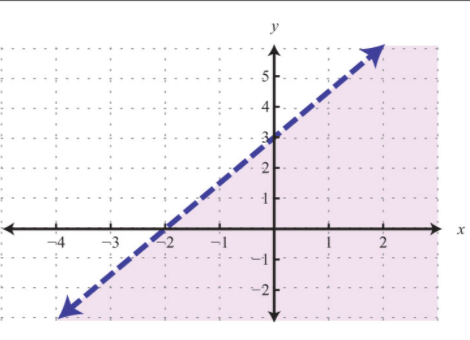Symbols
Linear Equations
Basic Math Vocabulary
What is the image?
Name it in English!
100

X

What is multiplication?

100

x

What is the horizontal axis?

100

A statement that two expressions are equal (such as 8 + 3 = 11 or 2x – 3 = 7)

What is an equation?

100What is linear inequality (x is2.5)?

100

variable

variable

200What is greater than?

200

y

What is the vertical axis?

200

A quantity that can have any one of a set of values or a symbol that represents such a quantity

What is a variable?

200What is a linear equation?

200

solución

What is solution?

300What is greater than or equal to?

300

(x, y)

What are solution points for a linear equation?

300

Where the line crosses the x axis.

What is the x intercept?

300What is linear inequality greater than or equal to300

eje

What is axis?

400

* () x

What are symbols for multiplication?

400

These have several solutions that form a line on a graph.

What are linear equations?

400

Where the line crosses the y axis.

What is the y intercept?

400What is linear inequality less than or equal to?

400

What is inequality?

500

m, b

What is slope and y-intercept of the line?

500

Solutions

What are sets of numbers that can satisfy an equation?

500

A statement that one expression or number is greater than or less than another

(such as 8 + 4 > 11 or 2x – 3 < 7)

What is inequality?

500What is linear inequality less than?

500

pendiente

What is slope?

Click to zoom